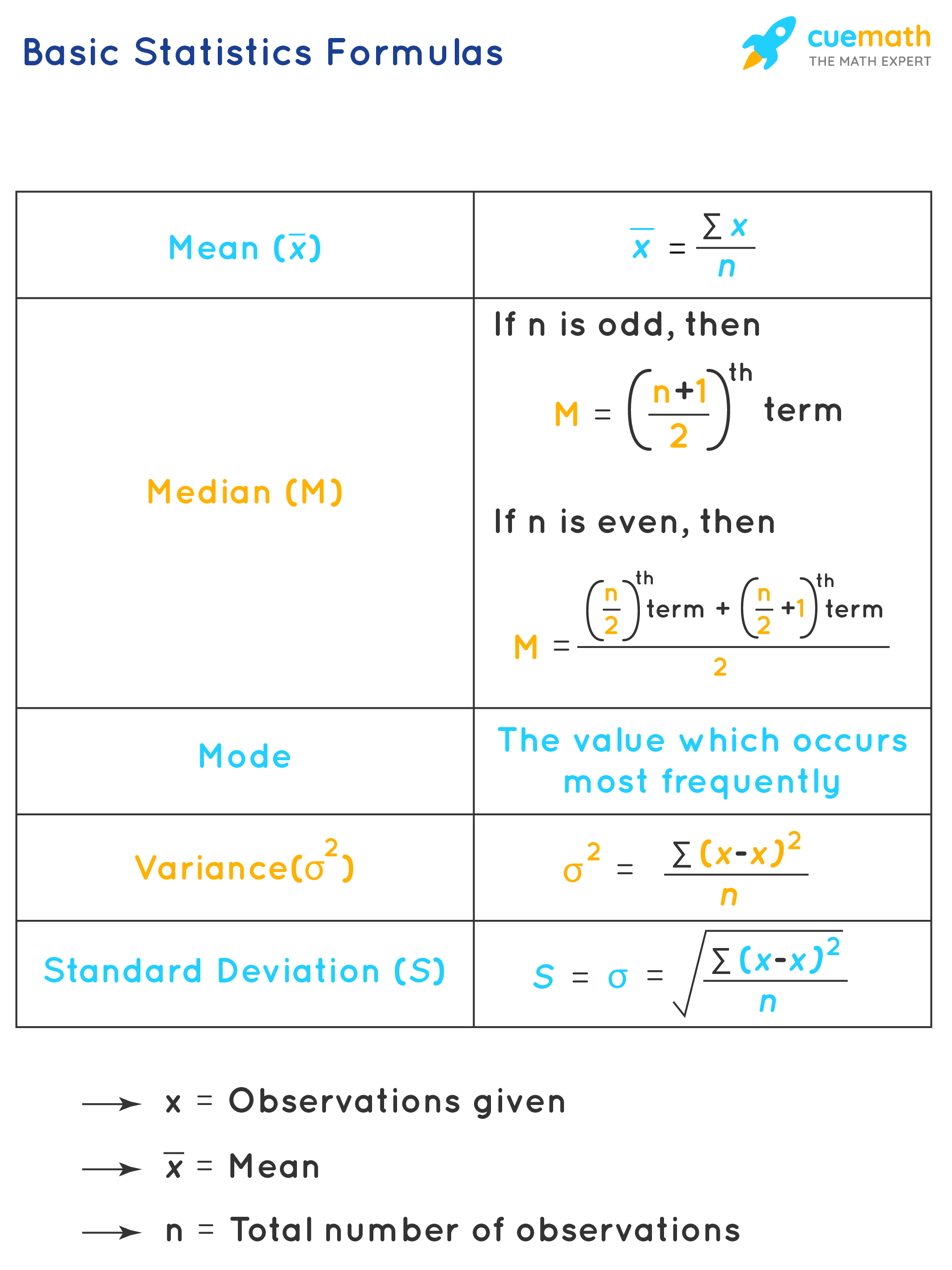# Basic Statistics Formula

Statistics is a branch of mathematics that deals with numbers and analysis of the data. Statistics is the study of the collection, analysis, interpretation, presentation, and organization of data.

In short, Statistics deals with collecting, classifying, arranging, and presenting collected numerical data in simple comprehensible ways. Statistics interprets various results from it and forecast possibilities. There are various basic statistics formulas to help us in dealing with facts, observations, and information which are in the form of numeric data only. With the help of statistics, we are able to find various measures of central tendencies and the deviation of data values from the center. Let us learn the basic statistics formulas along with a few solved examples.

## What Are the Basic Statistics Formulas?

The important statistics formulas are listed in the chart below:

$$\begin{array}{|c|c|} \hline \text { Mean } (\bar{x}) & \bar{x}=\frac{\sum x}{n} \\ \hline \text { Median (M)} & \begin{array}{c} \text { If } \mathrm{n} \text { is odd, then } \\ \mathrm{M}=\left(\frac{n+1}{2}\right)^{t h} \text { term } \\ \text { If } \mathrm{n} \text { is even, then } \\ \mathrm{M}=\frac{\left(\frac{n}{2}\right)^{t h} \text { term }+\left(\frac{n}{2}+1\right)^{t h} \text { term }}{2} \end{array} \\ \hline \text { Mode } & \text { The value which occurs most frequently } \\ \hline \text { Variance }(\sigma^{2}) & \sigma^{2}=\frac{\sum(x-\bar{x})^{2}}{n} \\ \hline \text { Standarad Deviation } (S)& S=\sigma=\sqrt{\frac{\sum(x-\bar{x})^{2}}{n}} \\ \hline \end{array}$$

where,
x = Observations given
$$\bar{x}$$ = Mean
n = Total number of observations## Solved Examples Using Basic Statistics Formula

### Example 1: Age of students = {14,15,16,15,17,15,18} Find the mode.

Solution:

Since there is only one value repeating itself, it is a unimodal list.

\begin{align} \text{Mode} = \left \{ {15} \right \}\end{align}

### Find the standard deviation of their marks. (Take √23.1= 4.8)

Solution

N = 4

Sample Mean (X̄) = $$\dfrac{812+836+982+769}{4}$$ = 849.75

Variance = $$\dfrac{\sum^{N}_{i=1} (X_i - \bar{X})^2}{N}$$

=$$\dfrac{\sum^{4}_{i=1} (X_i - 849.75)^2}{4}$$

= $$\dfrac{(812 - 849.75)^2 + (836 - 849.75)^2 + (982 - 849.75)^2 + (769 - 849.75)^2}{4}$$ = 6406.1875

Standard Deviation = $$\sqrt{6406.1875}$$ = 80.039

Answer: Standard Deviation for this data is 80.039.

Learn from the best math teachers and top your exams

• Live one on one classroom and doubt clearing
• Practice worksheets in and after class for conceptual clarity
• Personalized curriculum to keep up with school